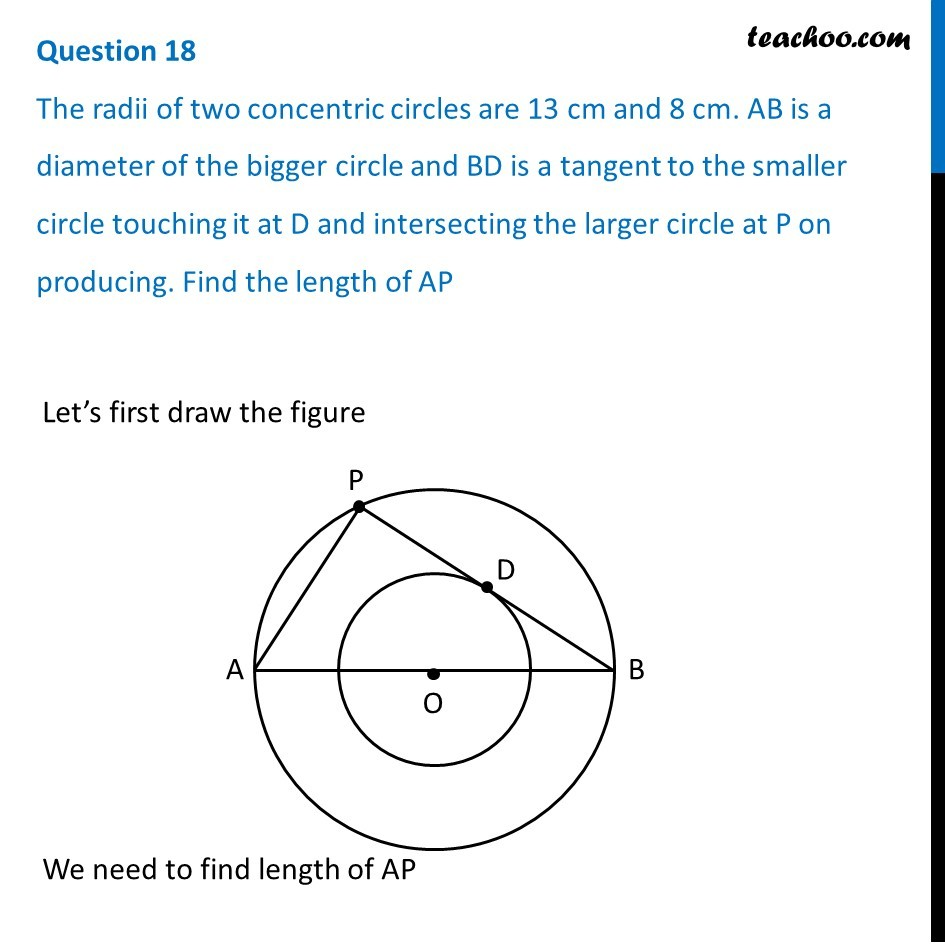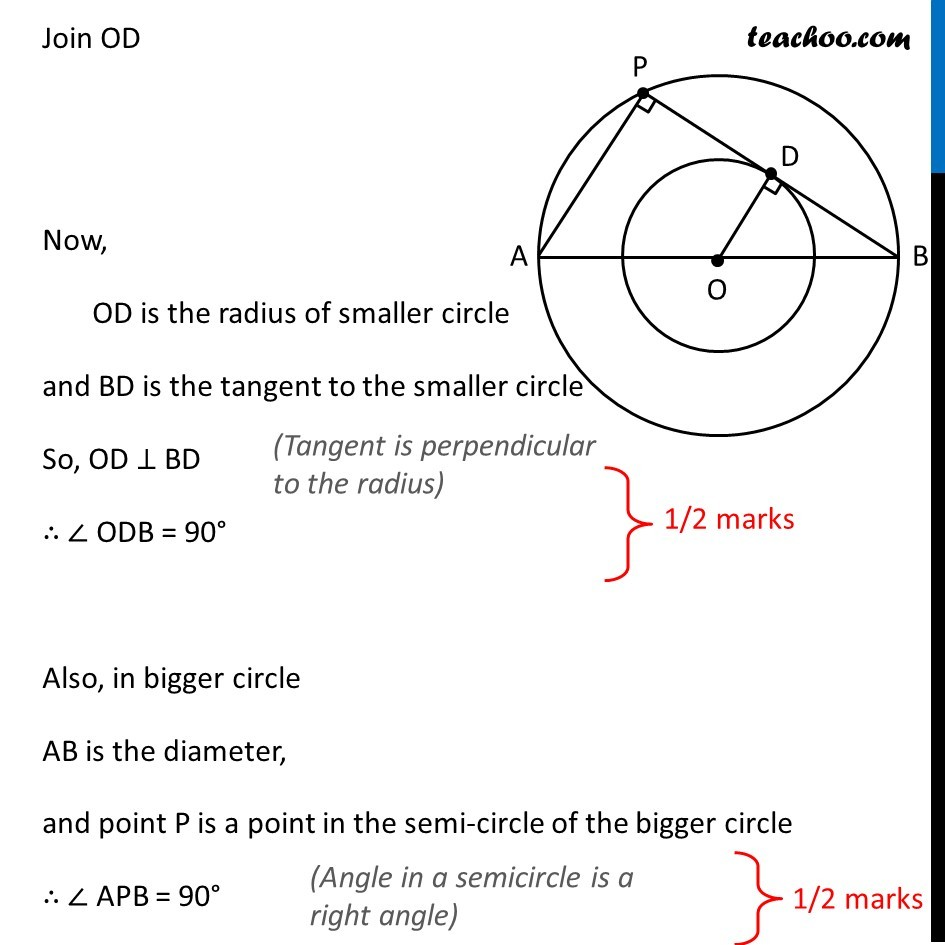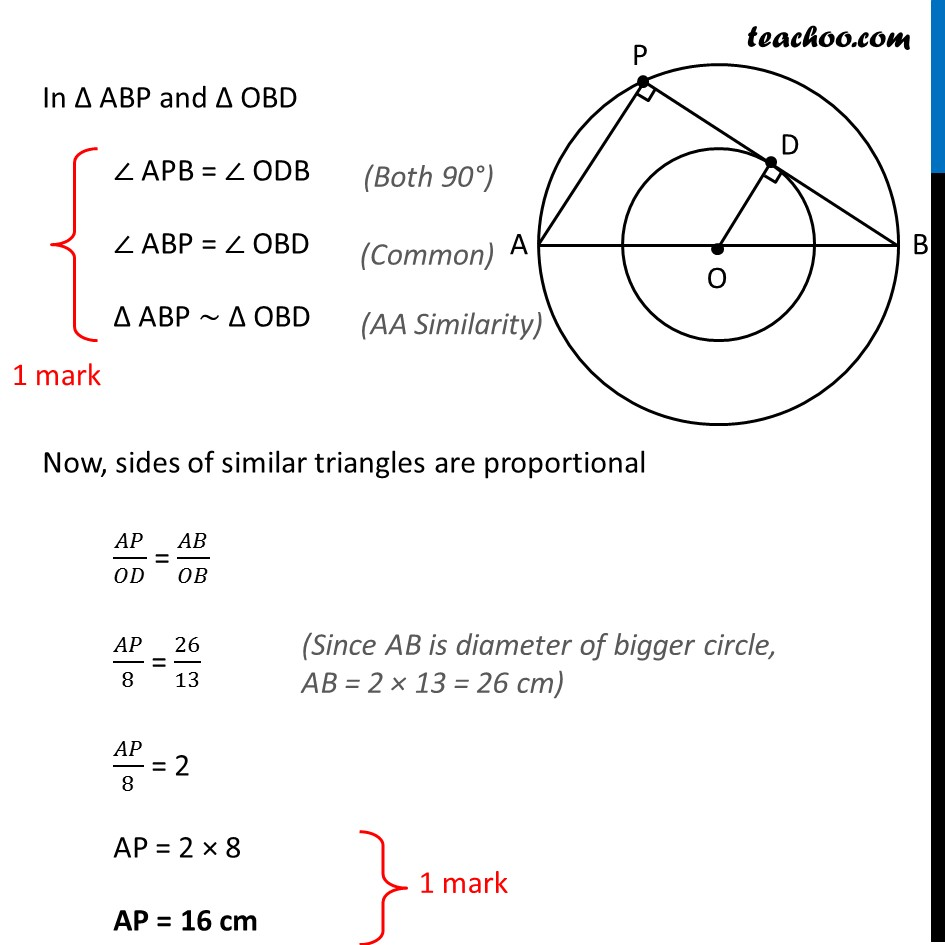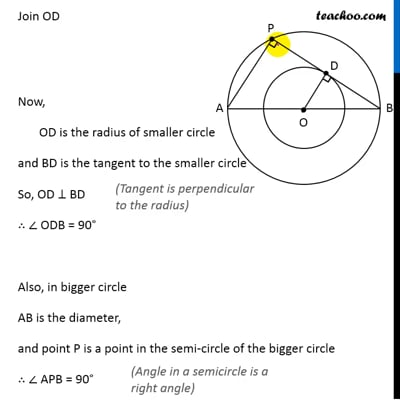CBSE Class 10 Sample Paper for 2019 Boards

Class 10
Solutions of Sample Papers for Class 10 Boards

Question 18

The radii of two concentric circles are 13 cm and 8 cm. AB is a diameter of the bigger circle and BD is a tangent to the smaller circle touching it at D and intersecting the larger circle at P on producing. Find the length of APThis video is only available for Teachoo black users

Learn in your speed, with individual attention - Teachoo Maths 1-on-1 Class

### Transcript

Question 18 The radii of two concentric circles are 13 cm and 8 cm. AB is a diameter of the bigger circle and BD is a tangent to the smaller circle touching it at D and intersecting the larger circle at P on producing. Find the length of AP Let’s first draw the figure We need to find length of AP Join OD Now, OD is the radius of smaller circle and BD is the tangent to the smaller circle So, OD ⊥ BD (Tangent is perpendicular to the radius) ∴ ∠ ODB = 90° Also, in bigger circle AB is the diameter, and point P is a point in the semi-circle of the bigger circle ∴ ∠ APB = 90° (Tangent is perpendicular to the radius) In Δ ABP and Δ OBD ∠ APB = ∠ ODB Both 90°) ∠ ABP = ∠ OBD (Common) Δ ABP ~ Δ OBD (AA Similarity) Now, sides of similar triangles are proportional 𝐴𝑃/𝑂𝐷 = 𝐴𝐵/𝑂𝐵 𝐴𝑃/8 = 26/13 (Since AB is diameter of bigger circle, AB = 2 × 13 = 26 cm) 𝐴𝑃/8 = 2 AP = 2 × 8 AP = 16 cm We need to find length of AP